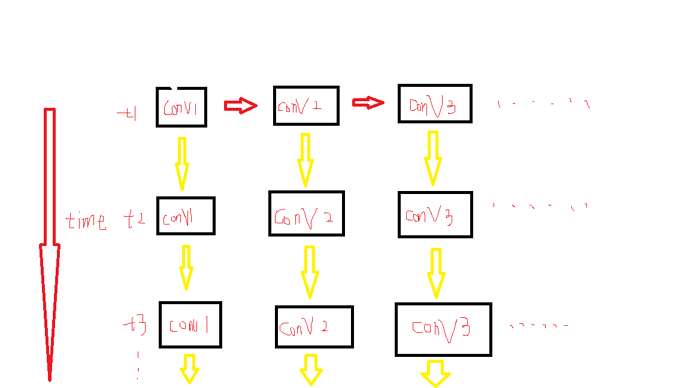# How to apply a simple model's parameters to a complicated model(two different model))I have written a simple model(only the horizontal part of above model) and trained the parameters. Now, I want to apply the simple model’s parameters to the above model(a different one). The complicated model can’t inherit from the simple one(or use simple one as a part), So,is there any way to do this work?

This will only work if each of the horizontal layers in your diagram are identical.

If so then you can save the trained model parameters:

``````torch.save(simple_model.state_dict(), "path/to/file.pth")
``````

and then load them into each of the horizontal layers:

``````filepath = "path/to/file.pth"

complex_model = [network() for i in range(num_layers)]

for layer in complex_model:
where `network` is the model you originally trained.